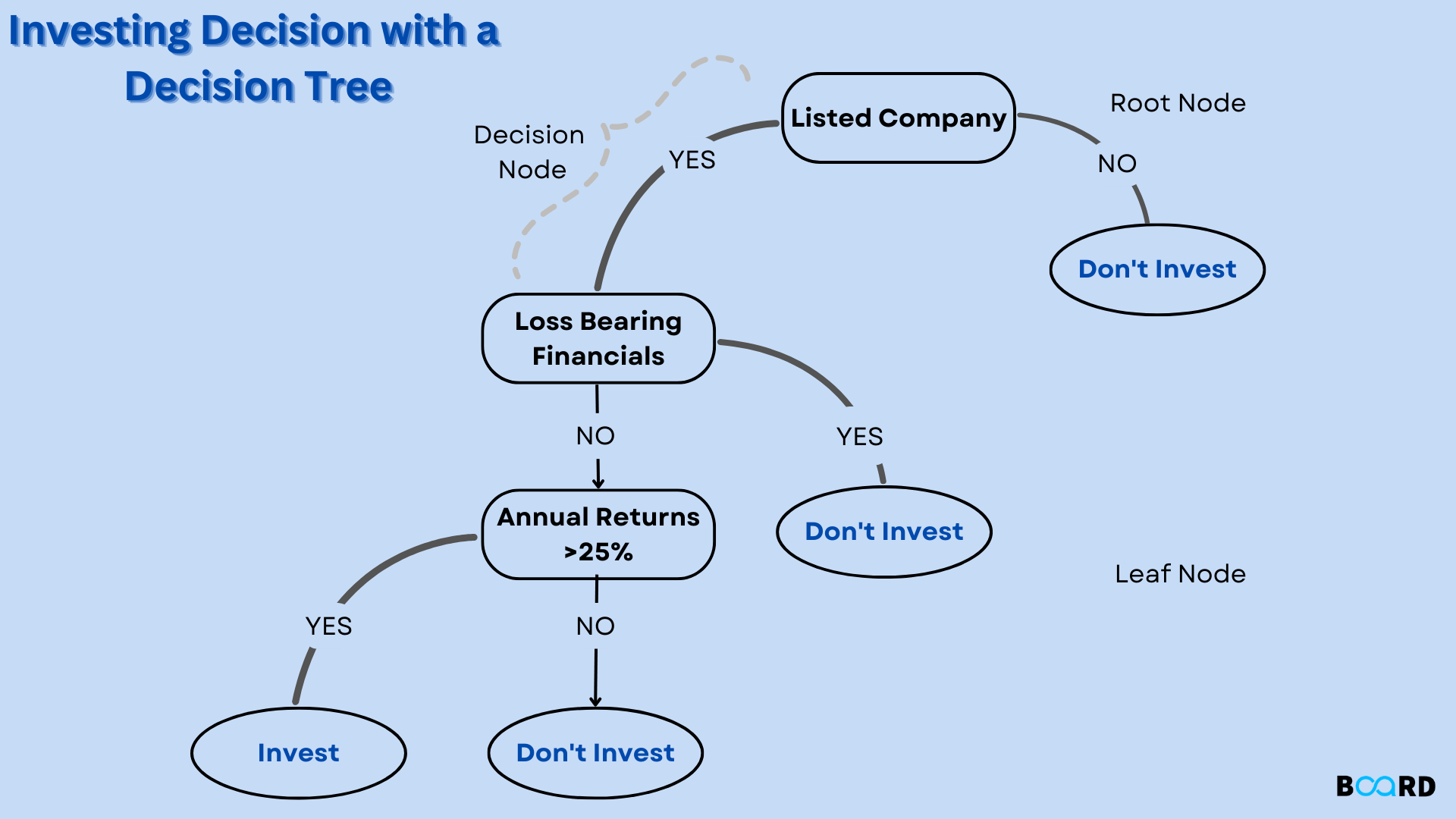# Decision Tree Algorithm: Explantation and Implementation## Introduction

The decision tree is the most efficient and popular categorization and prediction method. Each internal node in a decision tree represents a test on an attribute, each branch a test result, and each leaf node (terminal node) a class label. Decision trees are a sort of tree structure that mimics flowcharts.

## Construction of Decision Tree

A tree can be learned by segmenting the source set into subgroups based on an attribute value test. The process of repeating this operation on each derived subset is referred to as recursive partitioning. The recursion ends when the split no longer improves the predictions or when all members of a subset at a node have the same value for the target variable. Because it doesn't require parameter setting or domain knowledge, decision tree classifier development is perfect for exploratory knowledge discovery. Decision trees can be used to manage high-dimensional data. Classifiers using decision trees are frequently precise. Decision tree induction is a common inductive technique for learning classification information.

## Representation

Decision trees classify instances by placing them from the root to the leaf node of a tree, which indicates the instance's classification. As seen in the figure above, one must first test the attribute provided by the tree's root node before moving on to the branch of the tree that corresponds to the attribute's value in order to categorize an instance. The identical process is then applied once more to the subtree rooted at the new node. The decision tree in the above diagram assigns a classification to each leaf based on whether a certain morning is excellent for playing tennis and returns that classification. (in this case, either a Yes or a No).

## Gini Index

The Gini Index measures how exact a division is between the various categories that are identified. Using the Gini index, a score between 0 and 1 is evaluated, where 1 denotes a randomly distributed distribution of the components within classes. In this case, a low Gini index score is what we are aiming for. We'll use the Gini Index as the evaluation statistic to evaluate our decision tree model.

## Implementation

 from sklearn.datasets import make_classificationfrom sklearn import treefrom sklearn.model_selection import train_test_splitX, t = make_classification(100, 5, n_classes=2, shuffle=True, random_state=10)X_train, X_test, t_train, t_test = train_test_split( X, t, test_size=0.3, shuffle=True, random_state=1)model = tree.DecisionTreeClassifier()model = model.fit(X_train, t_train)predicted_value = model.predict(X_test)print(predicted_value)tree.plot_tree(model)zeroes = 0ones = 0for i in range(0, len(t_train)): if t_train[i] == 0: zeroes += 1 else: ones += 1print(zeroes)print(ones)val = 1 - ((zeroes/70)*(zeroes/70) + (ones/70)*(ones/70))print("Gini :", val)match = 0UnMatch = 0for i in range(30): if predicted_value[i] == t_test[i]: match += 1 else: UnMatch += 1accuracy = match/30print("Accuracy is: ", accuracy)
write your code here: Coding Playground

## Different Decisions Types

Categorical variable decision trees and continuous variable decision trees are the two basic forms of decision trees that are based on the target variable.

### Categorical Variable Decision Tree

A categorical variable decision tree has categorical target variables that are divided into categories. For example, the categories might be yes or no. Because of the categories, there are no intermediary steps in the decision-making process; all stages fall under one category.

### Continuous Variable Decision Tree

A decision tree with a continuous target variable is known as a continuous variable decision tree. For instance, depending on the information that is known about a person's occupation, age, and other continuous variables, it is possible to forecast their income.

## Application

• Evaluating potential chances for growth.
• Finding potential clients by using demographic information.
• Serving as a support tool in several fields.

## Summary

The supervised learning formulas family includes the choice tree algorithm. the choice tree technique, in distinction to alternative supervised learning ways, is capable of handling each classification and regression problem. By learning easy call rules derived from previous knowledge, a call Tree is employed to make a coaching model that will be accustomed predict the category or worth of the target variable (training data). In call trees, we start at the tree's root once anticipating a record's category label. we tend to distinction the foundation attribute's values thereupon of the attribute on the record. we tend to follow the branch related to that worth and progress to the subsequent node supported the comparison.